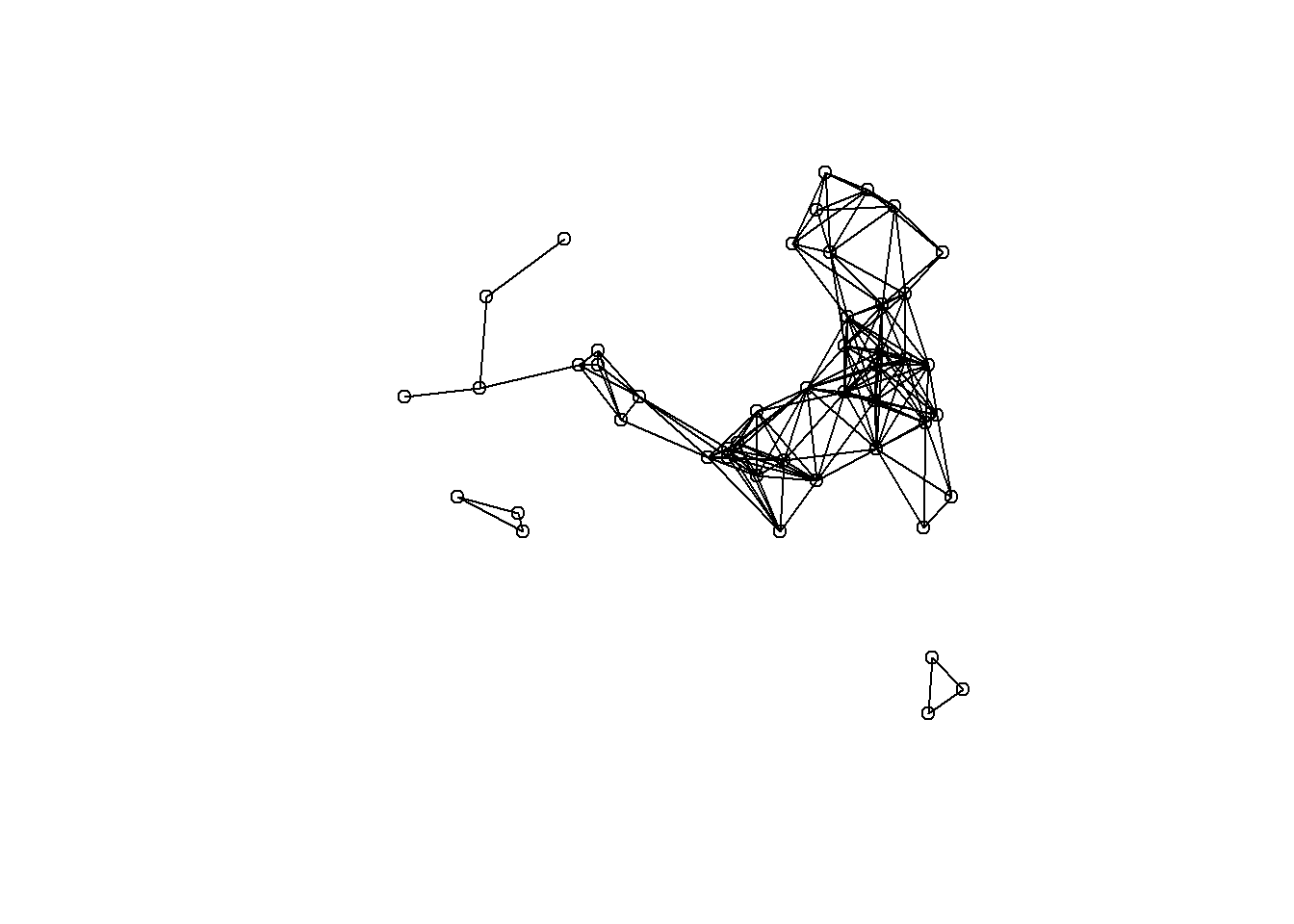This example walks you through the calculation of global and local Moran’s I and local Getis-Ord G* using the Maya terminal monument dates we discussed for the Premo (2004) article.

As we discussed, Moran’s I is a measure of the degree to which the value at a target site is similar to values at adjacent sites. Moran’s I is large and positive when the value for a given target (or for all locations in the global case) is similar to adjacent values and negative when the value at a target is dissimilar to adjacent values.

Getis-Ord G* identifies areas where high or low values cluster in space. G* is high where the sum of values within a neighborhood of a given radius or configuration is high relative to the global average and negative where the sum of values within a neighborhood are small relative to the global average and approaches 0 at intermediate values.

## Reading in data and plotting

The first step is to read in the table of Maya sites and dates and to create a Euclidean distance matrix defining the distance from every site to every other site in kilometers.

# load necessary packages
library(spdep)

# read file of Maya site locations and terminal dates

# create distance matrix in Km for all sites in 'maya'
maya.d <- as.matrix(dist(cbind(maya$EAST, maya$NORTH)))

As a first take on these data, we can plot the sites color coding them from yellow (early) to red (late) by terminal date.

# create vector of the order of sites by terminal date
ord <- order(maya$Date) # plot and color code sites, label by site number plot(maya[ord, 3:4], pch = 16, col = colorRampPalette(c("yellow", "red"))(nrow(maya)), xlab = "Km Easting", ylab = "Km Northing") text(maya[ord, 3:4], labels = maya[ord, 1], cex = 0.5, pos = 1)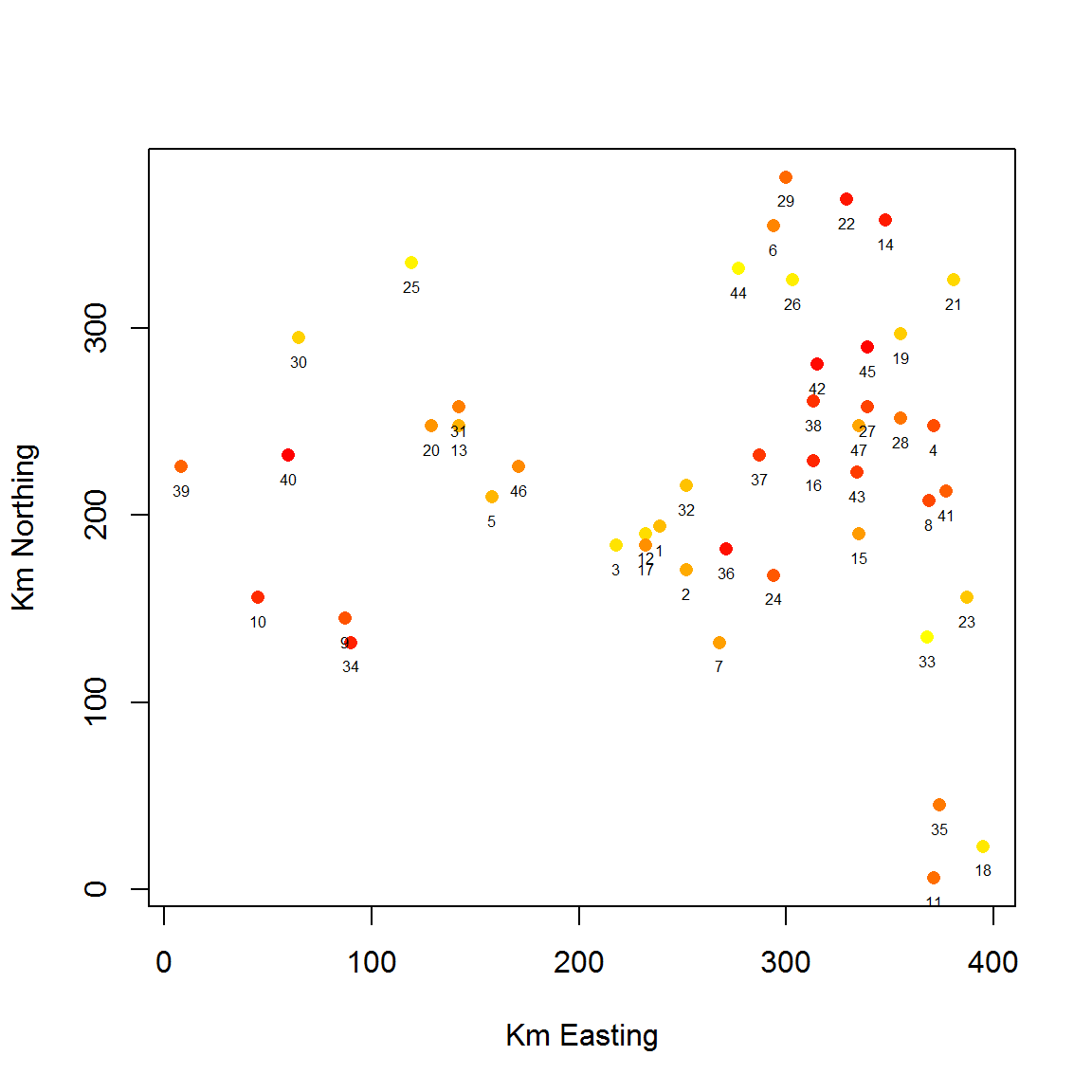Already there is a fair bit of spatial structure apparent in these data with late dates tending to cluster in the NE and W with the central zone characterized by earlier dates. ## Calculating the Global Moran’s I statistic and displaying a correlelogram Next we can calculate the global Moran’s I statistic to help us evaluate the strength of the apparent clustering we see in the map above. In order to calculate these statistics, we have to first convert the matrix of distances above to matrix of inverse weighted distances which we will simply call ‘w’. # create a matrix of weights based on the inverse distances between sites w <- 1/maya.d diag(w) <- 0 # calculate Moran's I statistic. The 'mat2listw' command simply converts the # distance matrix into a list of the form required by this command. moran.test(maya$Date, mat2listw(w))
##
##  Moran I test under randomisation
##
## data:  maya$Date ## weights: mat2listw(w) ## ## Moran I statistic standard deviate = 2.1941, p-value = 0.01411 ## alternative hypothesis: greater ## sample estimates: ## Moran I statistic Expectation Variance ## 0.055071568 -0.021739130 0.001225534 As the results above indicate, the value of Moran’s I is statistically significant suggesting spatial autocorrelation among terminal dates for the sites tested. It is also possible to evaluate variation in the Moran’s I statistic at varying distances to determine if a particular distance is characterized by a greater or lesser degree of spatial autocorrelation. Such a figure is referred to as a correlelogram. The convention of the ncf package used to plot the correlelogram here is that statistically significant Moran’s I values are shown as solid circles. # call required library library(ncf) # convert XY coordinates into coordinates object coord <- coordinates(maya[, 3:4]) # define correllogram at increments of 20 KM Date.I <- correlog(x = coord[, 1], y = coord[, 2], z = maya$Date, increment = 20,
resamp = 500, quiet = T)

# plot correllogram
plot(Date.I)
abline(h = 0, lty = 2)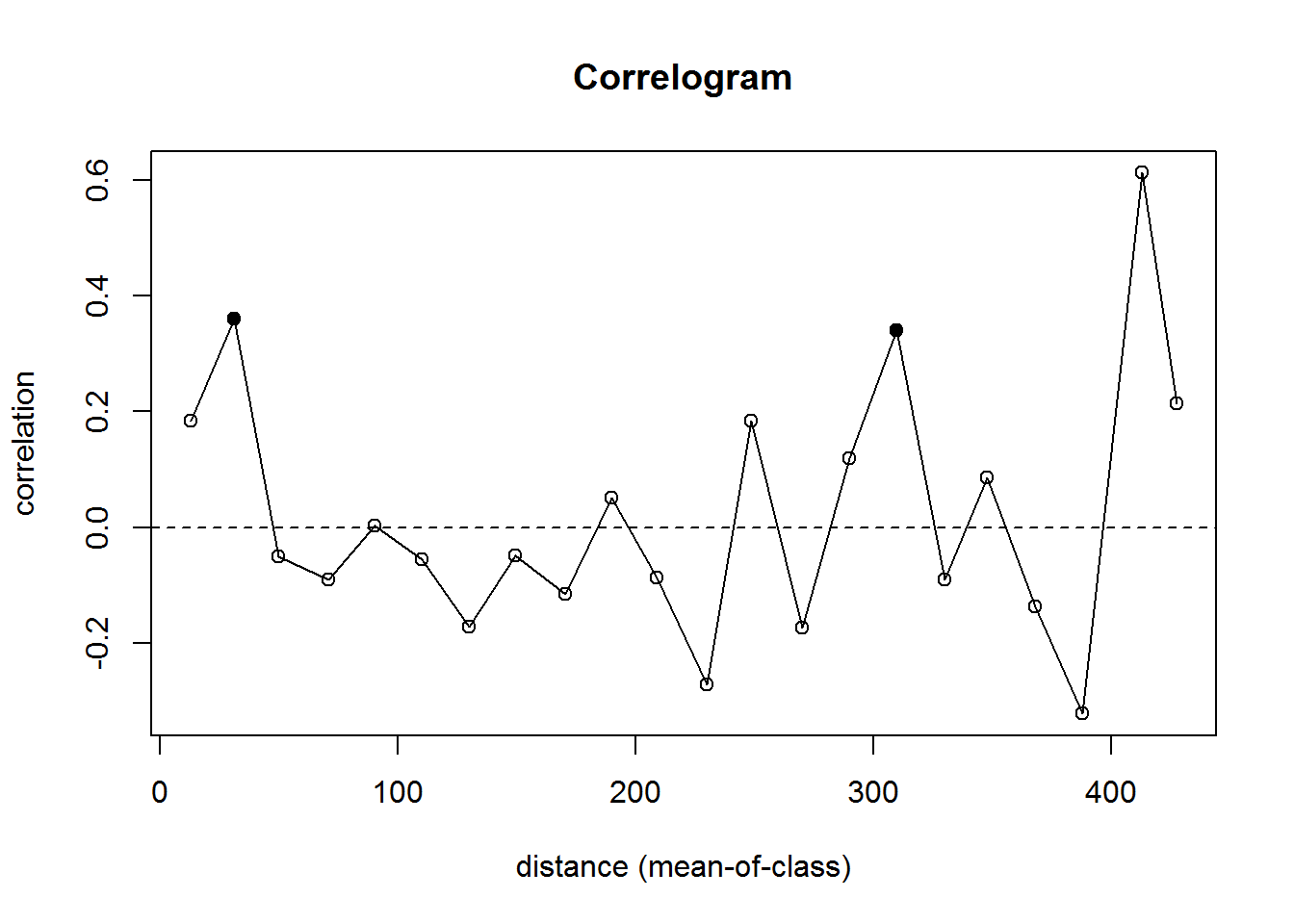As this plot illustrates, the terminal dates show positive autocorrelation at short distances, dates are close to neutral at moderate distances, but then show considerable variability at the furthest distances. As Premo (2004) suggests, however, this global statistic is not particularly informative (indeed it doesn’t tell us much that we couldn’t easily see by just looking at the map). Thus, he suggests the use of local Moran’s I along with the Getis-Ord G* statistic. I provide code for calculating both below.

## Calculating local Moran’s I and Getis-Ord G* statistics

The first step is to create a binary matrix defining neighborhoods for each distance where sites within the same neighborhood are coded as 1 and sites in different neighborhoods are coded as 0. Note that in the case of Moran’s I we want the diagonal of this matrix to be 0 as we do not want the site in question included in the calculation of the Moran’s I statistic. For G*, we want this diagonal to be defined as 1 as the site in question “counts” as a neighbor for that measure (see Premo 2004).

I show code for calculating Moran’s I and G* at one distance (25 Km) and then will provide the code for calculating these values at a range of distances.

# create new object from matrix of distances among sites to define weights
# (neighbors)
w25 <- maya.d
# define all distances greater than 25 Km as 0 (non-neighbors)
w25[w25 > 25] <- 0
# define the remaining values > 0 as 1 (neighbors from 1-25 Km)
w25[w25 > 0] <- 1

# calculate and display local Moran's I statistics at 25 Km
maya.M25 <- localmoran(maya$Date, mat2listw(w25)) head(maya.M25) # show the first several rows of output ## Ii E.Ii Var.Ii Z.Ii Pr(z > 0) ## 1 1.6983580 -0.06521739 2.9640194 1.024362116 0.15283213 ## 2 -0.8010565 -0.04347826 1.9700724 -0.539742259 0.70531260 ## 3 2.2872739 -0.06521739 2.9640194 1.366430364 0.08590196 ## 4 -0.0187592 -0.02173913 0.9751803 0.003017618 0.49879615 ## 5 0.2060258 -0.02173913 0.9751803 0.230645176 0.40879523 ## 6 NA 0.00000000 0.0000000 NA NA In the output above Ii = local Moran statistic, E.Ii = expected Moran statistic, Var.Ii = variance of the local Moran statistic, Z.Ii = the standard deviate of the local Moran statistic (this is probably what you want to use), and Pr() = the estimated p-value of the local Moran statistic. NAs are reported for any site that had no neighbors at the given lag distance. Next, I calculate G* at the same 25 Km distance. Note that to calculate G* (as opposed to G) we need to define the diagonal of the neighborhood matrix as 1, meaning that the target site is included in neighborhood calculations. Note that these scores differ slightly from those reported by Premo due to differences in the standardization procedure in the spdep package. # use the weight (neighbors) object from above but change the diagonal to 1 so # that the target site is included in calculations diag(w25) <- 1 # calculate and display local G* statistics at 25 Km maya.G25 <- as.matrix(localG(maya$Date, mat2listw(w25)))

head(maya.G25)  # show the first several rows of output
##            [,1]
## [1,] -1.6441580
## [2,]  0.3152465
## [3,] -1.6806084
## [4,]  0.4523900
## [5,] -0.7173647
## [6,] -0.2626174

The scores here are the standardized G* values by site. Note that, unlike Premo (2004), I have reported the cases where the only neighbor is the target site itself.

## Calculate local Moran’s I and G* at varying distances

In the next chunk of code, I demonstrate the procedures for calculating both local Moran’s I and G* at varying distances (25, 50, 75, 100, 125, 150, 175, and 200 km as used by Premo).Unfortunately, as we will see, Premo used a Visual Basic script for Microsoft Excel called “Rookcase” that had an error in a single line of code for calculations of local Moran’s I for everything but the first distance selected (I talked to Michael Sawada at the University of Ottawa who wrote the program and he was surprised anyone was still using his package but is in the process of writing and erratum). Thus, the results presented by Premo for everything but 25Km are incorrect and our results will differ. The next chunk calculates local Moran’s I and G* at distances between 25 and 200 Km at intervals of 25. The results are then compiled into a single matrix including only the standard deviates of Moran’s I and the standardized G* scores.

# define distance breaks between 25 and 200 at intervals of 25
dist.breaks <- seq(25, 200, by = 25)
# create matrix object to contain results
moran.out <- matrix(NA, nrow(maya), length(dist.breaks))
colnames(moran.out) <- c("M25", "M50", "M75", "M100", "M125", "M150", "M175", "M200")

# for every distance, define a weight (neighbors) matrix and calculate local
# Moran's I reporting the standard deviates
for (i in 1:length(dist.breaks)) {
w <- maya.d
w[w >= dist.breaks[i]] <- 0
w[w > 0] <- 1
moran.out[, i] <- localmoran(maya$Date, mat2listw(w), zero.policy = T)[, 4] } # create matrix object to contain results G.out <- matrix(NA, nrow(maya), length(dist.breaks)) colnames(G.out) <- c("G25", "G50", "G75", "G100", "G125", "G150", "G175", "G200") # for every distance, define a weight (neighbors) matrix and calculate local G* # reporting the standard deviates for (i in 1:length(dist.breaks)) { w <- maya.d w[w >= dist.breaks[i]] <- 0 w[w > 0] <- 1 diag(w) <- 1 #don't forget to change the diagonal to 1 G.out[, i] <- localG(maya$Date, mat2listw(w), zero.policy = T)
}

head(moran.out)  #show the first few rows of Moran's I values
##               M25       M50        M75        M100         M125
## [1,]  1.024362116 0.6965543 0.34240881  0.01029073 -0.009681183
## [2,] -0.539742259 0.6008448 0.33416393  0.08968550  0.248645203
## [3,]  1.366430364 1.7903920 1.32888562  0.87499148  0.212865869
## [4,]  0.003017618 0.4776147 1.17918198  0.97742172  0.753498047
## [5,]  0.230645176 0.5681445 0.88858599  1.12857760  0.206451263
## [6,]          NaN 0.2062898 0.02570768 -0.15745034 -0.188348601
##              M150       M175        M200
## [1,]  0.280606598  0.2598864 -0.17784803
## [2,] -0.007552889  0.2266268  0.28532658
## [3,] -0.632120713 -0.3559028  0.09403547
## [4,]  0.489057870  0.1526508  0.15265081
## [5,]  0.218598253 -0.1316244 -0.10338794
## [6,] -0.261756427 -0.2813404  0.09845494
head(G.out)  #show the first few rows of G* values
##             G25        G50         G75       G100        G125        G150
## [1,] -1.6441580 -1.2321860 -0.71522689 -0.1609477 -0.09067948 -0.72944653
## [2,]  0.3152465 -1.1357555 -0.71122226 -0.2863006 -0.57099333 -0.01968762
## [3,] -1.6806084 -2.0240672 -1.65655262 -1.2562805 -0.61631221  0.37203732
## [4,]  0.4523900  0.9500480  2.18020252  1.8998616  1.62414604  1.17767921
## [5,] -0.7173647 -1.0739208 -1.52121261 -1.9645688 -0.48916057 -0.51717740
## [6,] -0.2626174 -0.7113213 -0.04076636  0.8202540  1.08610076  1.58026645
##            G175       G200
## [1,] -0.7884485  0.8027572
## [2,] -0.6634513 -0.8987007
## [3,]  0.1184902 -0.7828250
## [4,]  0.4236990  0.4236990
## [5,]  0.2104980  0.2362632
## [6,]  1.9328959  0.0681004

## Plot maps of local Moran’s I and G*

Next we will plot the results for the 75 Km distance as bubble plots using the “bubble” command from the sp package. In these plots, positive values are shown in green and negative values are shown in purple with the bubble sized based on the scaled value of local I or G*

# create object for converstion to SpatialPointsDataFrame
maya.plot <- cbind(maya, moran.out, G.out)
# define EAST and NORTH as the coordinates
coordinates(maya.plot) <- c("EAST", "NORTH")

bubble(maya.plot, "M75", main = "Morans I at 75 Km")  #plot local Moran's I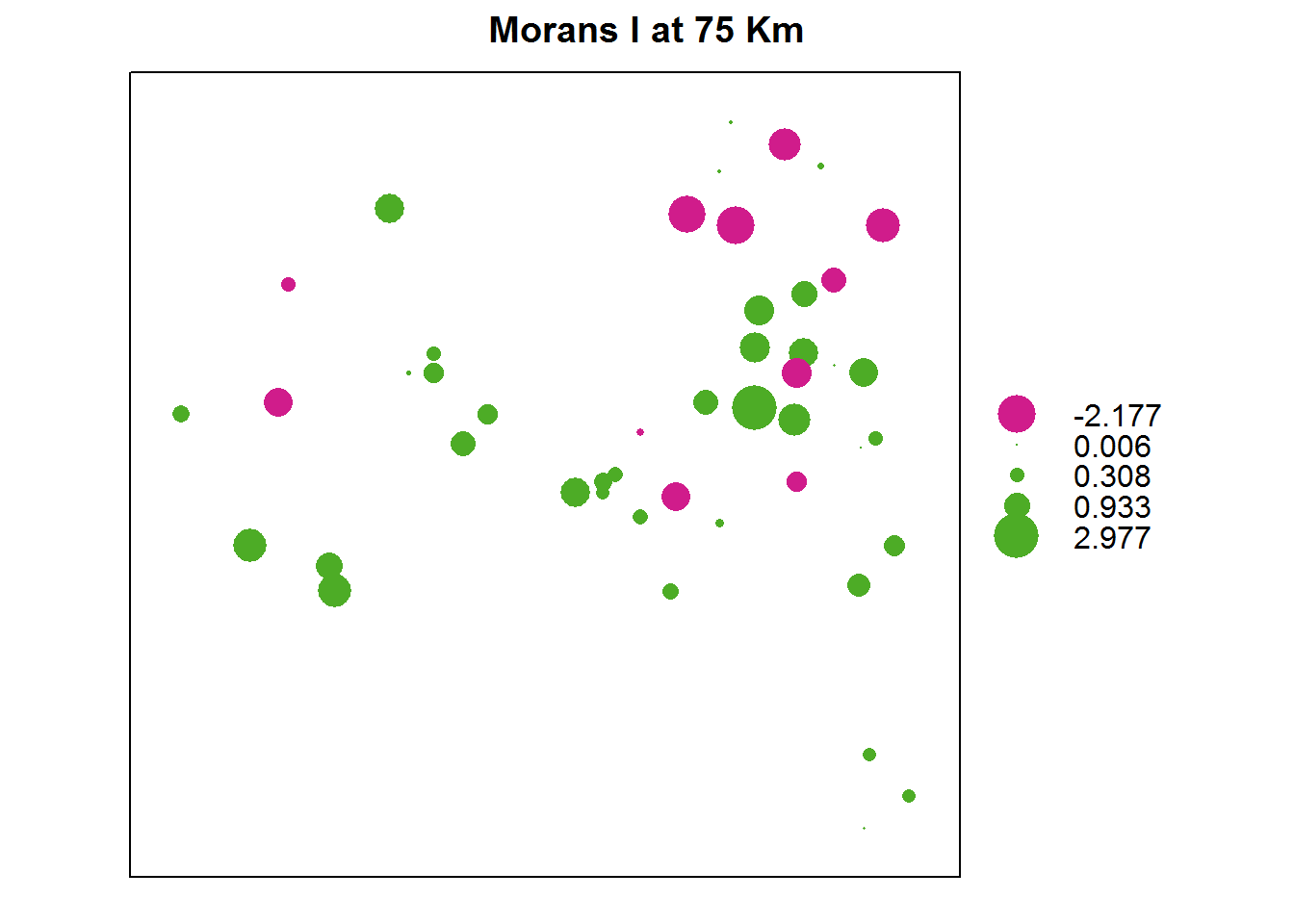bubble(maya.plot, "G75", main = "G* at 75 Km")  #plot local G*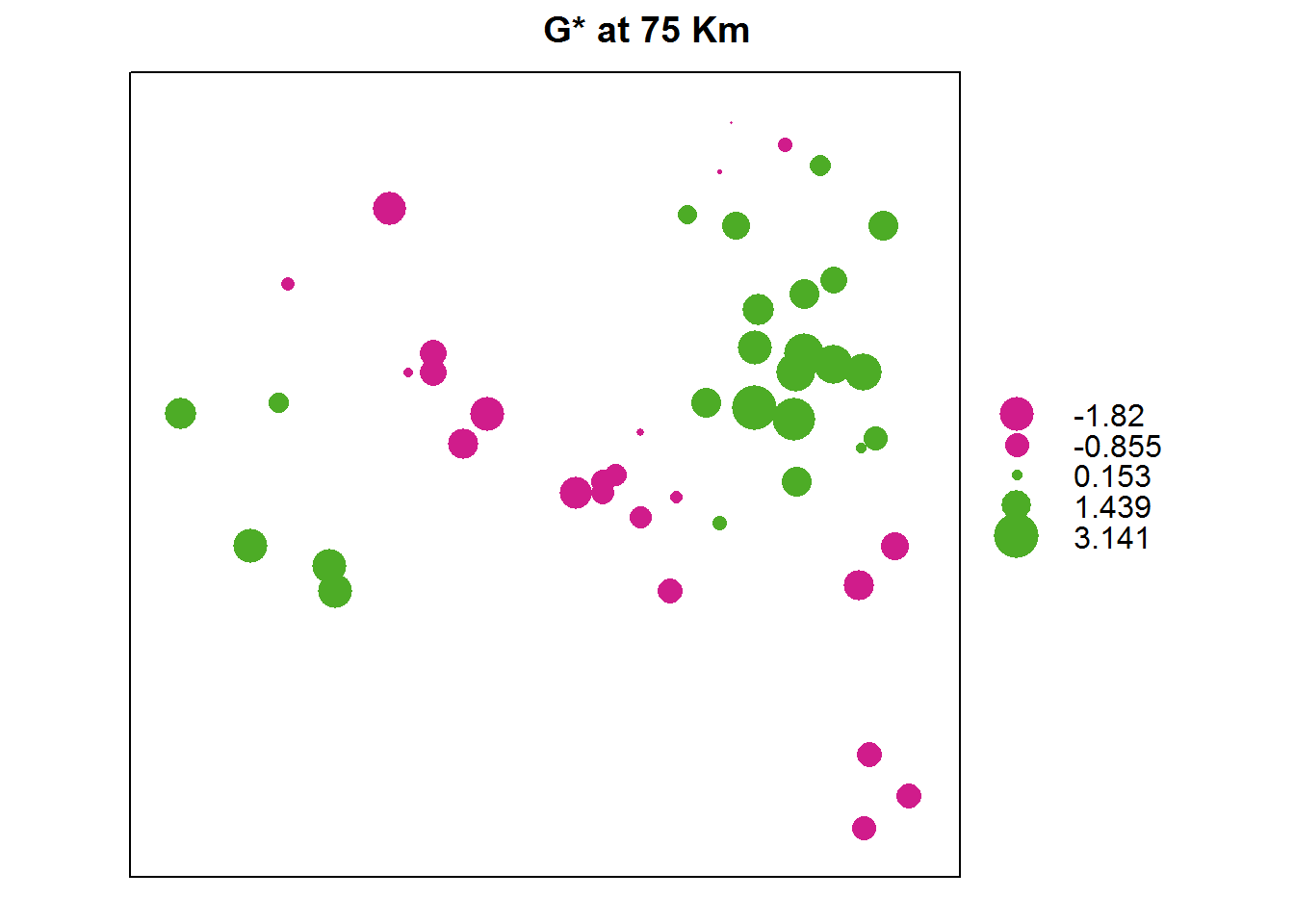Finally, if you would like to view the neighborhood definition at a given distance you can do that using a simple plot command.This command will display a network graph with points in their geographic location and edges drawn between sites in the same neighborhood. The example calculates the 75 Km neighborhood and then displays it.

w75 <- maya.d
w75[w75 > 75] <- 0
w75[w75 > 0] <- 1

plot(mat2listw(w75), coordinates(maya.plot))